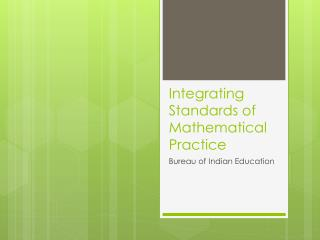DownloadDownload PresentationIntegrating Standards of Mathematical Practice

# Integrating Standards of Mathematical Practice

Télécharger la présentation## Integrating Standards of Mathematical Practice

- - - - - - - - - - - - - - - - - - - - - - - - - - - E N D - - - - - - - - - - - - - - - - - - - - - - - - - - -
##### Presentation Transcript

1. Integrating Standards of Mathematical Practice Bureau of Indian Education

2. The How and the What The Standards of Mathematical Practice describe varieties of expertise that mathematics educators at all levels should seek to develop in their students. These practices rest on important “processes and proficiencies” with longstanding importance in mathematics education.

3. Consistency With the CCSSM

4. Common Core Notes • They are NOT a rewrite of state standards • They are set to global standards • Most states were 1-2 years behind • Common Core is far more rigorous

5. Cultural responsiveness of Common Core What do standards of mathematical practice have in common with Native American pedagogy?

6. Commonality of Common Core Ojibwe Pedagogy Standards of Math Practice

7. The Standards • Make sense of problems and persevere in solving them • Reason abstractly and quantitatively • Construct viable arguments and critique reasoning • Model with mathematics • Use appropriate tools strategically • Attend to precision • Look for and make use of structure • Look for and express regularity in repeated reasoning www.corestandards.org

9. The Standards • Make sense of problems and persevere in solving them • Reason abstractly and quantitatively • Construct viable arguments and critique reasoning • Model with mathematics • Use appropriate tools strategically • Attend to precision • Look for and make use of structure • Look for and express regularity in repeated reasoning www.corestandards.org

10. Look for and express regularity in repeated reasoning • Children notice similarities in problems • Children create “shortcuts” • Children understand place value • Children use and understand invented algorithms for larger numbers

11. Make sense of problems and persevere in solving them • Children don’t rely on teacher for solution • Predictions to problems are reasonable • Children recall information correctly • Children can repeat question • Children don’t give up easily • Children ask for harder problems

12. Reason abstractly and quantitatively • Children can explain what numbers mean • Children can write equations to problems • Children can match numbers and objects • Children understand operations • Children use inverse operations • Children use multiple solution strategies

13. Construct viable arguments and critique the reasoning of others • Children can prove their answer • Children can disprove other answers • Children can identify counterexamples • Children use mathematical vocabulary • Children make accurate predictions • Children can explain another’s solution

14. Model with mathematics • Children make statements such as, “that’s like…” or “hey we did this before!” • Children can choose an operation that matches a problem • Children can connect formal and informal notation • Children can create a story problem

15. Use appropriate tools strategically • Children use many manipulatives • Children frequently draw math pictures • Children have math journals • Children can explain a solution by showing what the did with manipulatives or drawings • Children use and understand metric and standard rulers

16. Attend to precision • Children can use own words to define math concepts • Children use math vocabulary to describe a solution • Children are often asked to explain their solutions to class • Teachers commonly rephrase thinking • Teachers create many opportunities for children to share thinking

17. Look for and make use of structure • Children understand inverse and relative operations • Children use math facts to derive solutions • Children notice numerical relationships • Children use base-10 knowledge

18. School Data

19. 27% proficient in 2010 90% proficient in 2011 Grades 3-6